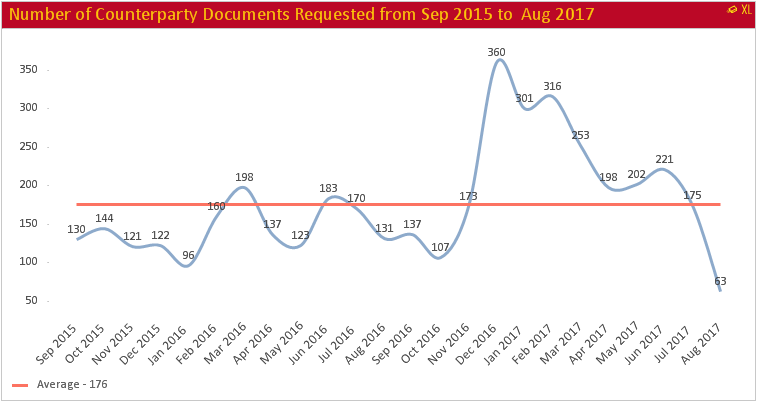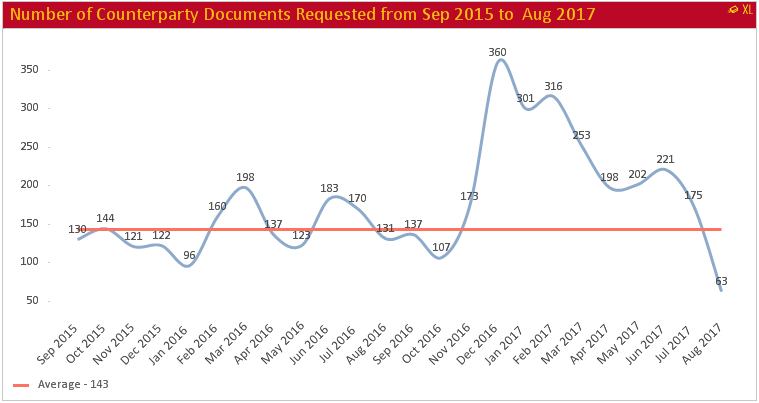# QlikView App Dev

Discussion Board for collaboration related to QlikView App Development.

Announcements
Action-Packed Learning Awaits! QlikWorld 2023. April 17 - 20 in Las Vegas: REGISTER NOW
cancel
Showing results for
Did you mean:Specialist II

## Two line charts with set analysis are behaving differently when a selection is made on the chart

Dimension

=MonthName([ReqDt])

Expression for Line Chart

=Count(
{<
[ReqDt]={'>=\$(=vBegDateLast24thMonth)<=\$(=vEndDateThisMonth)'},
[MDT]={'Val1', 'Val2'},
[SDT]={'Val3', 'Val4'}
>}
[ID])

Expression for Average Line Chart

=Round(Avg(TOTAL Aggr(Count(
{<
[ReqDt]={'>=\$(=vBegDateLast24thMonth)<=\$(=vEndDateThisMonth)'},
[MDT]={'Val1', 'Val2'},
[SDT]={'Val3', 'Val4'}
>}
[ID]), [ReqDt Date Year], [ReqDt DateMonth])))

Chart when no selection is madeChart when some month selection is done from chart

This image is showing month selectionThis image is showing resulting chartI'm trying to understand why Average Line chart reflects selection where as the other chart is still showing the original chart. Though both expressions (Line and Average)  are using same set analysis criteria.

Let me know if any additional information is needed.

Thanks & Regards,

Vijay

1 Solution

Accepted SolutionsMVP

The second one isn't using the same criteria.... for them to use the same criteria, you need set analysis in the outer aggregation as well

=Round(Avg(TOTAL

{<
[ReqDt]={'>=\$(=vBegDateLast24thMonth)<=\$(=vEndDateThisMonth)'},
[MDT]={'Val1', 'Val2'},
[SDT]={'Val3', 'Val4'}
>}

Aggr(Count(
{<
[ReqDt]={'>=\$(=vBegDateLast24thMonth)<=\$(=vEndDateThisMonth)'},
[MDT]={'Val1', 'Val2'},
[SDT]={'Val3', 'Val4'}
>}
[ID]), [ReqDt Date Year], [ReqDt DateMonth])))

2 RepliesMVP

The second one isn't using the same criteria.... for them to use the same criteria, you need set analysis in the outer aggregation as well

=Round(Avg(TOTAL

{<
[ReqDt]={'>=\$(=vBegDateLast24thMonth)<=\$(=vEndDateThisMonth)'},
[MDT]={'Val1', 'Val2'},
[SDT]={'Val3', 'Val4'}
>}

Aggr(Count(
{<
[ReqDt]={'>=\$(=vBegDateLast24thMonth)<=\$(=vEndDateThisMonth)'},
[MDT]={'Val1', 'Val2'},
[SDT]={'Val3', 'Val4'}
>}
[ID]), [ReqDt Date Year], [ReqDt DateMonth])))Specialist II
Author

Hi Sunny,

As always thanks for your prompt and useful response.

Thanks & Regards,

VijayCommunity Browser